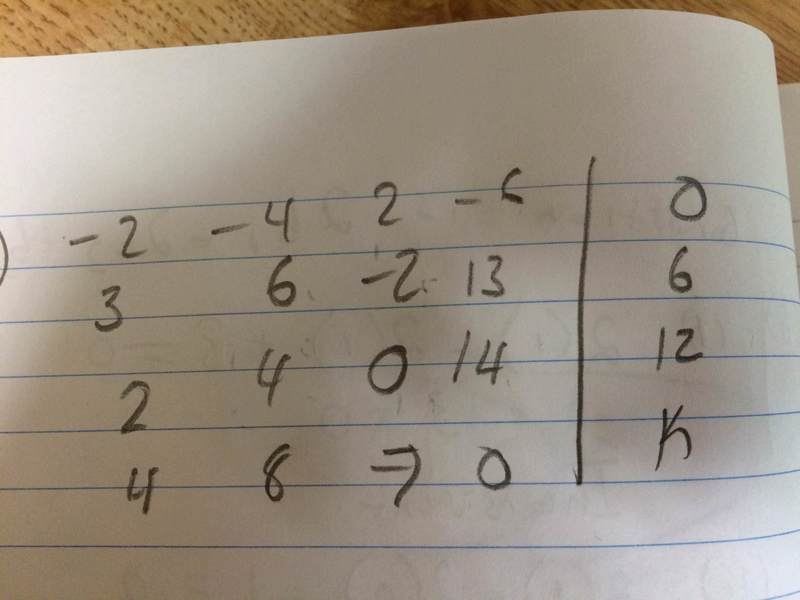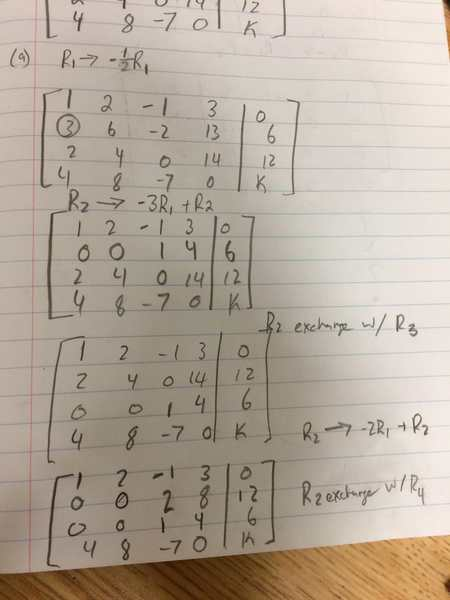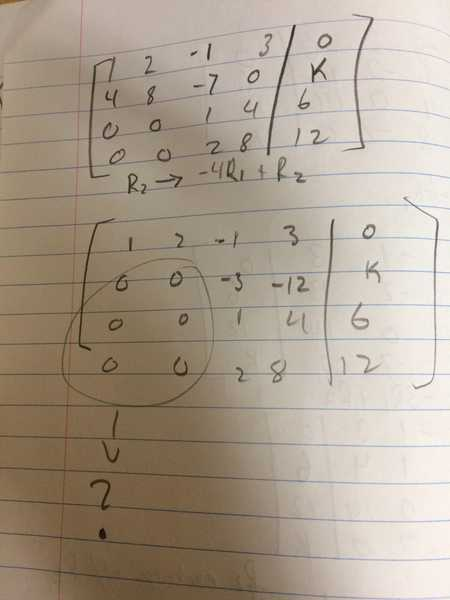# Reducing a matrix to echelon form

## Homework Statement(i) Reduce the system to echelon form C|d
(ii) For k = -12, what are the ranks of C and C|d? Find the solution in vector form if the system is consistent.
(iii) Repeat part (b) above for k = −18

## Homework Equations

Gaussian elimination I used here:
http://mathworld.wolfram.com/GaussianElimination.html

## The Attempt at a Solution[/B]

I've attempted reducing the matrix but have run into this problem where I have two zeroes leading in the last three rows. I don't know what I am doing wrong, so any help would be greatly appreciated.

I can't continue with either parts b or c without solving part a, and I'm not too sure about those parts either.

#### Attachments

I've attempted reducing the matrix but have run into this problem where I have two zeroes leading in the last three rows. I don't know what I am doing wrong, so any help would be greatly appreciated.
Your algebra looks ok. Having these zeros does not necessarily mean its wrong, and it is probably the reason that the problem is asking for the rank of the matrix in part ii.

Your algebra looks ok. Having these zeros does not necessarily mean its wrong, and it is probably the reason that the problem is asking for the rank of the matrix in part ii.
Alright, I understand. However, how would the ranks change regardless of my k value considering that the ranks only depend on the C part of C|d. Also, when it says to find the solution in vector form, does that mean I just write down the last column when I plug in k?

However, how would the ranks change regardless of my k value considering that the ranks only depend on the C part of C|d.
The rank of ##C## will not change. I am not completely sure what the question means when it asks for the rank of ##C|d##.
when it says to find the solution in vector form, does that mean I just write down the last column when I plug in k?
You can write the solution vector as the last column ##d## if you get the augmented matrix into reduced echelon form. Otherwise, you can perform Gaussian elimination to find the solution.